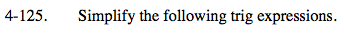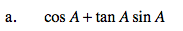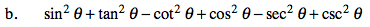### Home > PC > Chapter 4 > Lesson 4.3.1 > Problem4-125

4-125.
1. Simplify the following trig expressions. Homework Help ✎

1. cos A + tan A sin A

2. sin2 θ + tan2 θ − cot2 θ + cos2 θ − sec2 θ + csc2 θ1. Change all terms to sine and cosine.
2. Get a common denominator.
3. Use the Fundamental Pythagorean Identity.
4. Simplify.Use the Pythagorean Identities.
Change their forms to get 1s.

cos2θ + sin2θ = 1
1 + tan2θ = sec2θ
1 + cot2θ = csc2θ